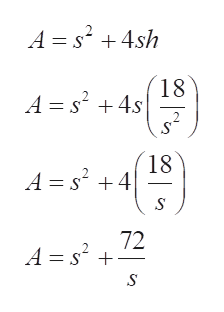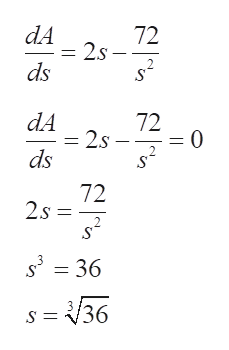An open box with a square base is to have a volume of 18 ft3Find the box dimensions that minimize the amount of material used. (Round your answers to two decimal places.)

Question

An open box with a square base is to have a volume of 18 ft3

Find the box dimensions that minimize the amount of material used. (Round your answers to two decimal places.)

Step 1

Consider the thickness of the material to be negligible.

Since the base is square shaped, the length and width of the box would be same as the side of the base.

Let the side of the base be s ft and height be h ft.

Given the volume of the box is 18ft3.

Volume of the box is given by s2h.

Hence,

Step 2

The material used is minimum when the surface area would be minimum.

The surface area of an open box is given by:

Plugging the value of h:help_outlineImage TranscriptioncloseA =s24sh 18 A ss S 2 18 A s24 72 A s2 fullscreen
Step 3

A function has a maximum or minimum value at a point where the first derivative is 0.

Applying derivative for the functio...help_outlineImage TranscriptionclosedA 72 2s s2 ds dA 72 2s = 0 2 ds 72 2s 2 3 36 s 36 fullscreen

Want to see the full answer?

See Solution

Want to see this answer and more?

Our solutions are written by experts, many with advanced degrees, and available 24/7

See Solution
Tagged in

Calculus